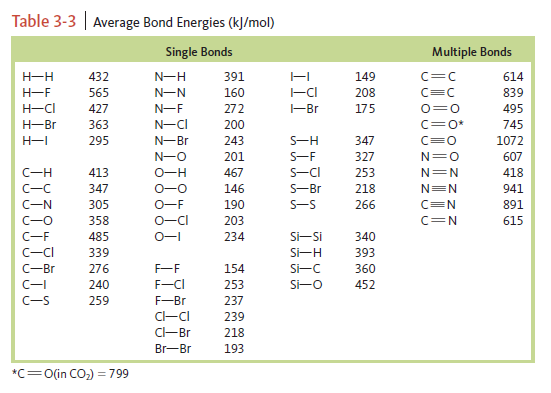# Problem: Consider the following reaction:H2O(g) + Cl 2O(g) → 2HOCl(g)     K 298 = 0.090For Cl2O(g),       ΔG°f = 97.9 kJ/mol       ΔH°f = 80.3 kJ/mol           S° = 266.1 J/K • mola. Calculate ΔG° for the reaction using the equation ΔG° = -RT ln(K).b. Use bond energy values (Table 3-3) to estimate ΔH° for the reaction.c. Use the results from parts a and b to estimate ΔS° for the reaction.d. Estimate ΔH°f and S° for HOCl(g).e. Estimate the value of K at 500. K.

###### FREE Expert Solution
100% (378 ratings)
###### Problem Details

Consider the following reaction:

H2O(g) + Cl 2O(g) → 2HOCl(g)     K 298 = 0.090

For Cl2O(g),
ΔG°f = 97.9 kJ/mol
ΔH°f = 80.3 kJ/mol
S° = 266.1 J/K • mol

a. Calculate ΔG° for the reaction using the equation ΔG° = -RT ln(K).
b. Use bond energy values (Table 3-3) to estimate ΔH° for the reaction.c. Use the results from parts a and b to estimate ΔS° for the reaction.

d. Estimate ΔH°f and S° for HOCl(g).

e. Estimate the value of K at 500. K.